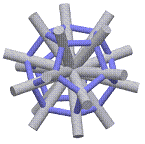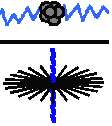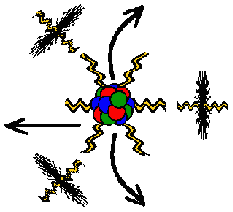Properties of Energy & Matter
By James Clifford Cranwell 4/19/98

## Part IV Mass

In an atom, most of the Mass is contained in the nucleus. However, an Electron does have mass and the Proton/Electron mass ratio is 1836.1527556561 ,As a result of this division we have canceled the force of light/gravity on all forty radii of the Electron-Proton combination in this example and we are left with the number of times the Electron shell (inanimate or inert unit Flux) is contained in the 18 curled radii of the Proton (that's right...mass has to be something and the only something there is...is Flux). Now we divide 1836.1527556561 by 10Ö26 (the exact value without the cancelled speed of light and gravity portion...negative one times speed of light, these were cancelled in the initial division) then divide by 18 (the number of curled radii) and get the result 2.0005510109034 This means everyone's measurements are correct to within five / ten thousandths and we know there are two Flux taken into the curl of every radii as matter forms.

Quantum Weirdness:
If there were only two objects in the universe, an observer and the Earth, both completely weightless/massless, the observer would be tricked into thinking he/she has weight because of the gravitational tug and mass because of the large concentration of gravitational tug, in their reality there are only forces. The Mass of the actual Flux particle is negligible... it is substantial only when it has a vibration or a gravitational pull on it.Therefore Mass is only a vibration or a pull...it isn't something we can actually take to the beach.Proof...
E = mc^2
(Energy equals Mass times the speed of light squared) and...
E = hf
(Energy equals frequency, h is constant). Therefore, we can replace E in the first equation with hf and get the result hf = mc^2 or drop the constants and we see that f = m (Frequency = Mass). When Mass is released as Energy it leaves the nucleus as Electrons traveling through free space at the speed of light...don't forget...the Electron is two dimensions so, Energy equals Mass traveling at the speed (or length) of light in two dimensions or E = mc^2
part Vhf = mc^2
f = mc^2/h
h = mc^2/f
Gc=1/(10(Ö26)-1) Goddess401Home Page